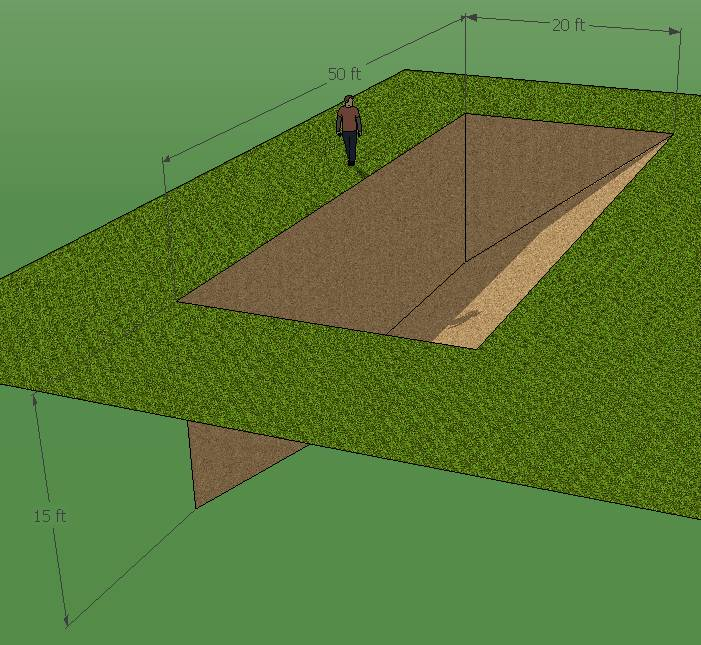SEARCH HOMEMath Central Quandaries & QueriesQuestion from Piero: I want to fill my backyard slope with fill dirt. The slope is 50 feet wide and the distance from the top of the top of the slope to the bottom is about 15 feet deep and the slope angle is at a 45. I want to know how many yards of dirt do I need to fill a space that is 20 feet out, 15 feet deep at a 45 degree angle.Hi Piero.

I think there is something strange with the numbers you've sent me. If it is a 45 degree angle, the depth (15 ft) must match the distance out (20 ft), but 15 is not the same as 20. That would be about 37 degrees.

There is an easy way to visualize this though.If you doubled the space exactly and rotated it around, you would have a rectangular box, whose volume (yards of dirt) is exactly twice what you need.So just punch those widths, depths and lengths into our volume calculator at http://mathcentral.uregina.ca/Volume and take half the answer.

Cheers,
Stephen La Rocque.Math Central is supported by the University of Regina and The Pacific Institute for the Mathematical Sciences.